# Solving nonlinear eigenvalue problems

## Introduction

We consider the problem of finding eigenvalues $\lambda\in\Sigma$ and nonzero eigenvectors $\mathbf{x}$ of a nonlinear eigenvalue problem (NLEP)

$A(\lambda) \mathbf x = \mathbf 0.$

Here $\Sigma$ is a compact target set in the complex plane and $A(\lambda)$ is a family of $n\times n$ matrices depending analytically on $\lambda$. A popular approach for solving such problems is to approximate $A(\lambda)$ by a polynomial or rational eigenvalue problem of the form

$Q_N(\lambda) = b_0(\lambda) D_0 + b_1(\lambda) D_1 + \cdots + b_N(\lambda) D_N,$

where the $D_j$ are $n\times n$ matrices, and the $b_j$ are polynomials or rational functions in $\lambda$. Provided that the $b_j$ can be generated by a linear recursion, the problem $Q_N(\lambda)\mathbf{x} = \mathbf 0$ can be "linearised" into a linear pencil $(A_N,B_N)$ of size $Nn \times Nn$, which in practice can be rather large depending on $N$ and $n$.

## The NLEIGS linearisation

The NLEIGS linearisation  is based on rational interpolation, with the $b_j$ chosen as rational basis functions of the form

$\displaystyle b_0(\lambda) = 1, \quad b_{j+1}(\lambda) = \frac{(\lambda-\sigma_j)}{\beta_{j+1}(1-\lambda/\xi_{j+1})} b_j(\lambda).$

Here, the $\sigma_j$ are interpolation nodes on the boundary of $\Sigma$, and the $\xi_j$ are poles which can be chosen freely, for example all at infinity (which leads to a polynomial interpolant) or on a singularity set $\Xi$ of $A(\lambda)$. The advantage of employing an interpolation-based approximation $Q_N(\lambda)$ is that the matrices $D_j$ can be obtained solely by sampling $A(\sigma_j)$, provided that the nodes $\sigma_j$ are distinct. For more details we refer to [3, Section 2.1].

The numbers $\beta_j$ are scaling factors which, as suggested in , are chosen so that $\displaystyle \max_{\lambda\in\Sigma} |b_j(\lambda)| \approx 1.$ This scaling has the advantage that the norms $\| D_j \|_F$ give an indication of the approximation accuracy of $Q_N(\lambda)$ for $A(\lambda)$; see [3, Section 4] for more details.

## Leja-Bagby sampling

To obtain a computationally efficient method it is desirable that $Q_N(\lambda) \approx A(\lambda)$ is a good approximation for all $\lambda\in\Sigma$ with a small degree parameter $N$. This suggests to use an (asymptotically) optimal rational interpolation procedure and we propose to choose the $(\sigma_j,\xi_j)$ as Leja-Bagby points on $(\Sigma,\Xi)$ [1, 9].

More precisely, choosing $\sigma_0\in\Sigma$ arbitrarily, we define the nodes $\sigma_j$ and poles $\xi_j$ so that the following conditions are satisfied:

$\displaystyle \max_{\lambda\in\Sigma} |s_j(\lambda)| = |s_j(\sigma_{j+1})|, \quad \min_{\lambda\in\Xi} |s_j(\lambda)| = |s_j(\xi_{j+1})|,$

with the nodal functions $s_j$ defined as $\displaystyle s_j(\lambda) = \frac{(\lambda - \sigma_0)\cdots (\lambda-\sigma_j)}{(\lambda - \xi_1)\cdots (\lambda-\xi_j)}.$

## The Gun problem

We now demonstrate the above with an example taken from the problem collection . We use the so-called gun problem, which is a model of a radio-frequency gun cavity . The corresponding NLEP is

$\displaystyle A(\lambda)\mathbf x = [K-\lambda M + i\sqrt{\lambda} W_1 + i\sqrt{\lambda-108.8774^2} W_2]\mathbf x = \mathbf 0,$

where $K, M, W_1, W_2$ are sparse $9956\times 9956$ matrices. Let us define a function handle to the NLEP.

if exist('nlevp') ~= 2
disp(['http://www.maths.manchester.ac.uk/our-research/research-groups' ...
'/numerical-analysis-and-scientific-computing/numerical-analysis/' ...
'software/nlevp/'])
return
end

[coeffs, fun] = nlevp('gun');
n = size(coeffs{1}, 1);
A = @(lam) 1*coeffs{1} - lam*coeffs{2} + ...
1i*sqrt(lam)*coeffs{3} + ...
1i*sqrt(lam-108.8774^2)*coeffs{4};


The target set $\Sigma$ for this problem is an upper-half disk with center $62500$ and radius $50000$. Note that the definition of $A(\lambda)$ involves two branch cuts $(-\infty,0]$ and $(-\infty,108.8774^2]$ caused by the square roots, and the union of these two is a good choice for the singularity set $\Xi$.

We can now use the utility function util_nleigs to sample the NLEP on the target set $\Sigma$ using poles from the singularity set $\Xi$. The function requires as inputs a function handle to $A(\lambda)$, the vertices of $\Sigma$ and $\Xi$ represented by polygons, a tolerance for the sampling procedure, and the maximal number of terms.

Nmax  = 50;
Sigma = 62500 + 50000*exp(1i*pi*[1, linspace(0, 1)]);
Xi   = [-inf, 108.8774^2];
tol  = 1e-15;
QN   = util_nleigs(A, Sigma, Xi, tol, Nmax);
disp(QN)

	RKFUNM object of size 9956-by-9956 and type (32, 31).
Complex sparse coefficient matrices of size 9956-by-9956.
Complex-valued Hessenberg pencil (H, K) of size 33-by-32.


As we can see, the output of util_nleigs is an RKFUNM object QN representing $Q_N(\lambda)$, a rational matrix-valued function which interpolates $A(\lambda)$ at the nodes $\sigma_j$ (i.e., $Q_N(\sigma_j) = A(\sigma_j)$ for all $j=0,1,\ldots,N$). We can evaluate $Q_N$ at any point $z$ in the complex plane by typing QN(z). The linearize function can be used to convert $Q_N$ into an equivalent linear matrix pencil structure $L_N(z) = A_N - z B_N$ with the same eigenvalues as $Q_N$. Via $L_N$ we can also access the norms $\|D_j\|_F$ of the matrices $D_j$ in the expansion of $Q_N$. Apparently, a degree of $N=32$ was sufficient to represent $A(\lambda)$ to accuracy $\mathrm{tol} = 10^{-15}$:

LN   = linearize(QN);
figure(1), semilogy(0:LN.N, LN.nrmD/LN.nrmD(1), 'r-'), grid on
legend('relative Frobenius norm of D_j'); xlabel('j')
axis([0, LN.N, 1e-16, 1])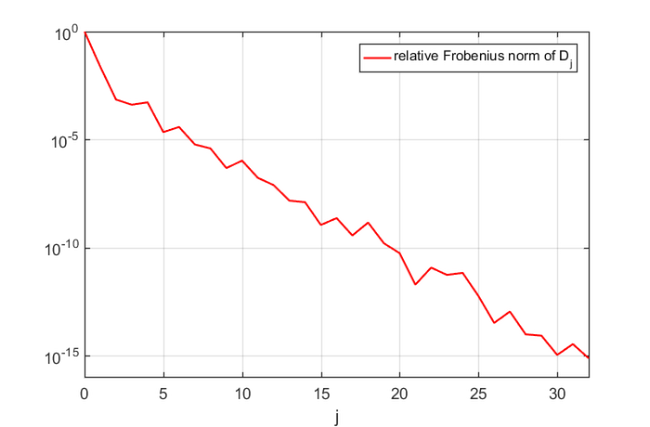Luckily this $N=32$ is quite small due to the Leja-Bagby sampling strategy employed by util_nleigs. However, the full linearisation matrices $(A_N,B_N)$ are of size $Nn\times Nn$ and hence quite large. Here is a spy plot of $(A_N,B_N)$.

[AN,BN] = LN.get_matrices();
figure(2)
subplot(1,2,1), spy(AN), title('A_N')
subplot(1,2,2), spy(BN), title('B_N')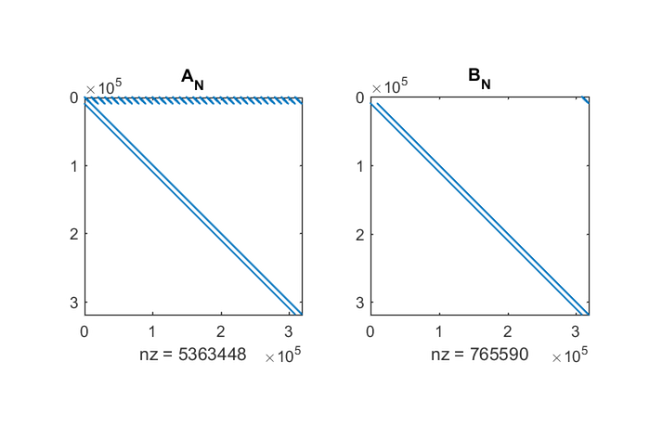The LN structure provides two function handles multiply and solve, which can be used by the rat_krylov function to compute a rational Krylov basis for $(A_N,B_N)$ without forming these matrices explicitly [7, 8]. For the rational Arnoldi algorithm we choose, rather arbitrarily, $5$ cyclically repeated shifts in the interior of $\Sigma$.

shifts = [9.6e+4, 7.9e+4+1.7e+4i, 6.3e+4, 4.6e+4+1.7e+4i, 2.9e+4];


Let us first plot the target set, the sampling points $\sigma_j$, the poles $\xi_j$, and the shifts of the rational Krylov space:

figure(3)
fill(real(sqrt(Sigma)), imag(sqrt(Sigma)), [1 1 .6])
hold on
plot(sqrt(LN.sigma), 'gx', 'Color', [0 .5 0])
plot(sqrt(LN.xi(LN.xi>0))+1i*eps, 'r.', 'MarkerSize', 14)
plot(sqrt(shifts), 'mo')
xlabel('Re sqrt(lambda)'), ylabel('Im sqrt(lambda)')
legend('target set \Sigma', 'interpolation nodes \sigma_j', ...
'poles \xi_j', 'RK shifts', 'Location', 'NorthWest')
axis([0,350,-10,110])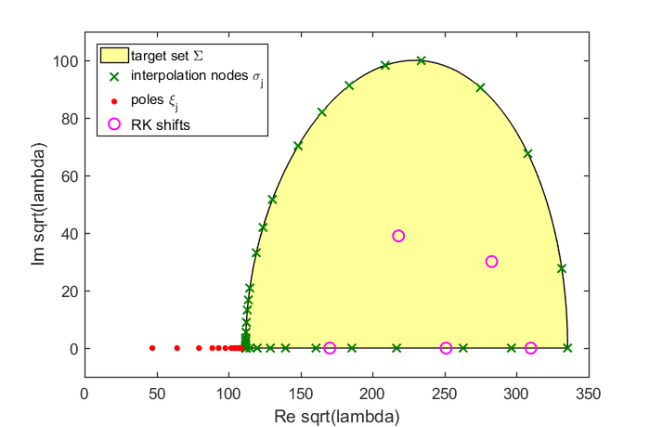The computation of the rational Arnoldi decomposition $A_N V_{m+1} \underline{K_m} = B_N V_{m+1} \underline{H_m}$ is conveniently performed by providing the pencil structure LN as the first input argument to the rat_krylov function. Here, $m=70$ and the starting vector is chosen at random.

Note: The shifts in this example are cyclically repeated and the solve function provided in the LN structure attempts to reuse LU factors of $n\times n$ matrices whenever possible. The five LU factors required for this example are stored automatically as persistent variables within the solve function.

v = randn(LN.N*n, 1);
shifts = repmat(shifts, 1, 14);
[V, K, H] = rat_krylov(LN, v, shifts, struct('waitbar', 1));


From the rational Arnoldi decomposition we can easily compute the Ritz pairs for the linearisation $(A_N,B_N)$. In the following we extract the Ritz values in the interior of $\Sigma$ and find, consistently with , that there are 21 Ritz values. The leading $n$ elements of the corresponding Ritz vectors (normalised to unit norm) are then approximations to the eigenvectors $\mathbf{x}$ of $A(\lambda)$.

[X, D] = eig(H(1:end-1, :), K(1:end-1, :));
ritzval = diag(D);
ind = inpolygon(real(ritzval), imag(ritzval), ...
real(Sigma), imag(Sigma));
ritzval = ritzval(ind);
ritzvec = V(1:n, 1:end)*(H*X(:, ind));
ritzvec = ritzvec/diag(sqrt(sum(abs(ritzvec).^2)));
disp(length(ritzval))

    21


Let us compute the nonlinear residual norm $\| A(\lambda)\mathbf{x}\|_2$ for all 21 Ritz pairs $(\lambda,\mathbf{x})$:

res = arrayfun(@(j) norm(A(ritzval(j))*ritzvec(:, j), 'fro'), ...
1:length(ritzval));

figure(4)
semilogy(res, 'b-o'), xlim([1, length(res)])
legend('residual norm of Ritz pairs')
xlabel('index of Ritz pair'), hold on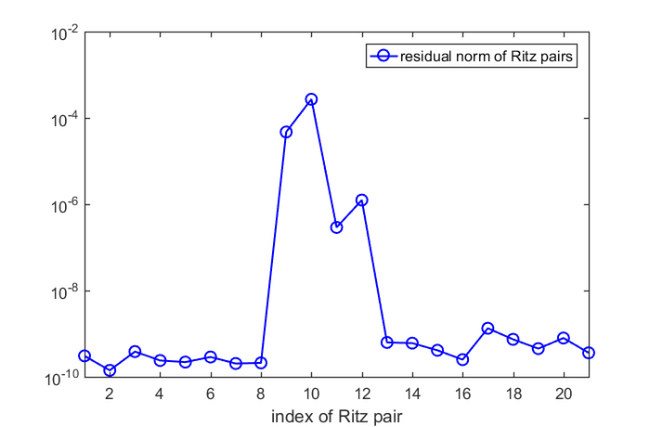We find that all but $4$ Ritz pairs are good approximations to the eigenpairs of the nonlinear problem. Let us run five more rational Arnoldi iterations by using as shift the mean of the four nonconverged Ritz values. This can be done by simply extending the existing rational Arnoldi decomposition.

% Use mean of Ritz values.
shifts = repmat(mean(ritzval(res>1e-8)), 1, 5);
% Extend the decomposition.
[V, K, H] = rat_krylov(LN, V, K, H, shifts, struct('waitbar',1));


Now let us compute the improved Ritz pairs and the corresponding nonlinear residuals exactly as above:

[X, D] = eig(H(1:end-1, :), K(1:end-1, :));
ritzval = diag(D);
ind = inpolygon(real(ritzval), imag(ritzval), ...
real(Sigma),   imag(Sigma));
ritzval = ritzval(ind);
ritzvec = V(1:n, 1:end)*(H*X(:, ind));
ritzvec = ritzvec/diag(sqrt(sum(abs(ritzvec).^2)));

res = arrayfun(@(j) norm(A(ritzval(j))*ritzvec(:, j), 'fro'), ...
1:length(ritzval));

figure(4)
semilogy(res, 'r-o')
legend('residual norm of Ritz pairs','residual norm (extended)')All wanted Ritz pair are now of sufficiently high accuracy and we are done. Finally, here is a plot of the Ritz values, which coincides with [3, Figure 4(a)]:

figure(3)
plot(sqrt(ritzval), 'b+')
legend('target set \Sigma', 'interpolation nodes \sigma_j', ...
'poles \xi_j', 'RK shifts', '21 Ritz values', ...
'Location', 'NorthWest')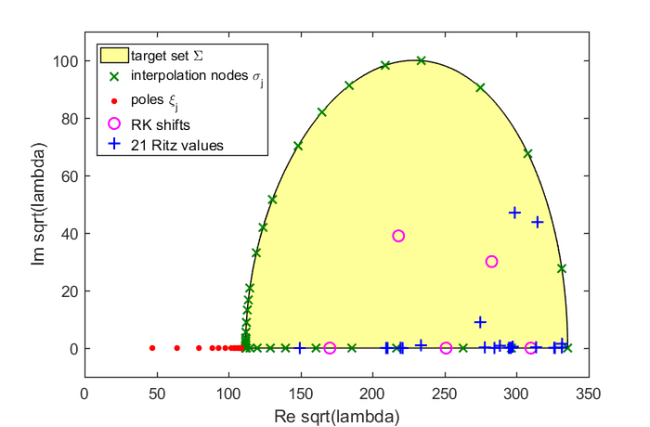## Variants and extensions

The linearisation computed by util_linearise_nlep is referred to as the "static variant of NLEIGS" in . The NLEIGS algorithm, which is also available online, supports the dynamic expansion of the linearisation $(A_N,B_N)$ as the rational Arnoldi iteration progresses. This dynamic expansion is inspired by the "infinite Arnoldi algorithm" presented in . The CORK algorithm in  is a memory-efficient variant of NLEIGS which exploits the special structure of the Krylov basis vectors $V_{m+1}$ associated with $(A_N,B_N)$.

## References

 T. Bagby. On interpolation by rational functions, Duke Math. J., 36:95--104, 1969.

 T. Betcke, N. J. Higham, V. Mehrmann, C. Schröder, and F. Tisseur. NLEVP: A collection of nonlinear eigenvalue problems, ACM Trans. Math. Software, 39(2):7:1--7:28, 2013.

 S. Güttel, R. Van Beeumen, K. Meerbergen, and W. Michiels. NLEIGS: A class of fully rational Krylov methods for nonlinear eigenvalue problems, SIAM J. Sci. Comput., 36(6):A2842--A2864, 2014.

 E. Jarlebring, W. Michiels, and Karl Meerbergen. A linear eigenvalue algorithm for the nonlinear eigenvalue problem, Numer. Math., 122(1):169--195, 2012.

 B.-S. Liao. Subspace Projection Methods for Model Order Reduction and Nonlinear Eigenvalue Computation, PhD thesis, Dept. of Mathematics, University of California at Davis, 2007.

 A. Ruhe. Rational Krylov algorithms for nonsymmetric eigenvalue problems, Recent Advances in Iterative Methods. Springer New York, pp. 149--164, 1994.

 A. Ruhe. Rational Krylov: A practical algorithm for large sparse nonsymmetric matrix pencils, SIAM J. Sci. Comput., 19(5):1535--1551, 1998.

 R. Van Beeumen, K. Meerbergen, W. Michiels. Compact rational Krylov methods for nonlinear eigenvalue problems, SIAM J. Matrix Anal. Appl., 36(2):820--838, 2015.

 J. Walsh. On interpolation and approximation by rational functions with preassigned poles, Transactions of the American Mathematical Society, 34:22--74, 1932.